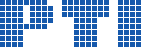Polish Information Processing Society# The density Turán problem for some 3-uniform unihypercyclic linear hypergraphs. An efficient testing algorithm

## Halina Bielak, Kamil Powroźnik

Citation: Proceedings of the 2015 Federated Conference on Computer Science and Information Systems, M. Ganzha, L. Maciaszek, M. Paprzycki (eds). ACSIS, Vol. 5, pages 563571 ()

Full text

Abstract. Let $\mathcal{H}=(V,\mathcal{E})$ be a $3$-uniform linear hypergraph with one hypercycle $\mathcal{C}\_3$. We consider a blow-up hypergraph $\mathcal{B}[\mathcal{H}]$.